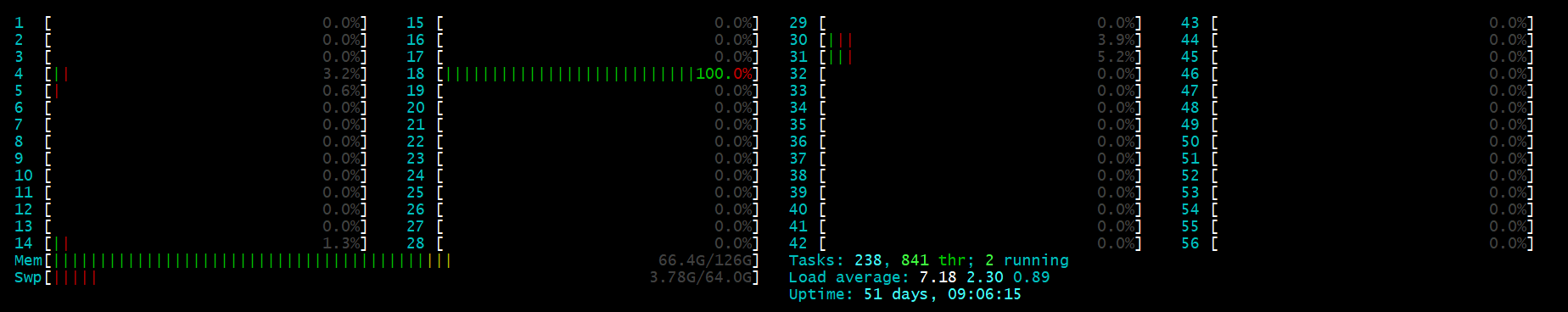# Python Multiprocessing多进程 使用tqdm显示进度条的实现

1.背景

2.函数要求

`pip install pathos`

```from pathos.multiprocessing import ProcessingPool as Pool
from tqdm import tqdm```

3.代码

```def F(X,lamda=10,weight=0.05):
res={}
res.update(F_1(X,lamda=lamda,weight=weight))
res.update(F_2(X,lamda=lamda,weight=weight))
return res```

x 是 F 的输出，是一个dict (字典格式)

```zip_lamda = [lamda for i in range(len(X)) ]
zip_weight = [weight for i in range(len(X)) ]
with tqdm(total=len(cold_sequences)) as t:
for i, x in enumerate(pool.imap(F,X,zip_lamda,zip_weight)):
X[i,:] = [x[key] for key in x.keys()]
Y[i,] = 0
t.update()
pool.close()
pool.join()```

4.结果

mutiprocess 加速前mutiprocess 加速后#### 您可能感兴趣的文章:

• 本文相关：
• python多进程池 multiprocessing pool用法示例
• python3多进程 multiprocessing 模块实例详解
• python多进程multiprocessing.pool类详解
• python多进程库multiprocessing中进程池pool类的使用详解
• python多进程并发(multiprocessing)用法实例详解
• python multiprocessing.manager介绍和实例（进程间共享数据）
• 详细介绍python进度条tqdm的使用
• python的tqdm模块的使用
• python开发之函数定义实例分析
• python中的break、continue、exit()、pass全面解析
• python3随机漫步生成数据并绘制
• python3使用matplotlib绘制条形图
• 使用python实现抓取腾讯视频所有电影的爬虫
• gauss-seidel迭代算法的python实现详解
• python2.7简单连接与操作mysql的方法
• pygame游戏之旅 计算游戏中躲过的障碍数量
• python批量修改文件夹及其子文件夹下的文件内容
• python通过ftplib登录到ftp服务器的方法
• 免责声明 - 关于我们 - 联系我们 - 广告联系 - 友情链接 - 帮助中心 - 频道导航